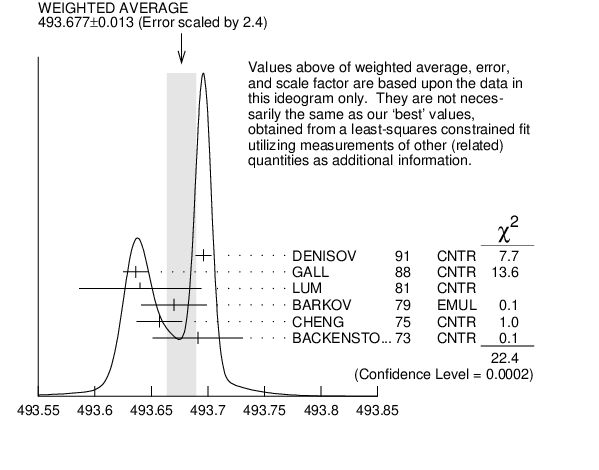# ${{\boldsymbol K}^{\pm}}$ MASS INSPIRE search

VALUE (MeV) DOCUMENT ID TECN CHG  COMMENT
$\bf{ 493.677 \pm0.016}$ OUR FIT  Error includes scale factor of 2.8.
$\bf{ 493.677 \pm0.013}$ OUR AVERAGE  Error includes scale factor of 2.4.
$493.696$ $\pm0.007$ 1
 1991
CNTR - Kaonic atoms
$493.636$ $\pm0.011$ 2
 1988
CNTR - Kaonic atoms
$493.640$ $\pm0.054$
 1981
CNTR - Kaonic atoms
$493.670$ $\pm0.029$
 1979
EMUL $\pm{}$ ${{\mathit e}^{+}}$ ${{\mathit e}^{-}}$ $\rightarrow$ ${{\mathit K}^{+}}{{\mathit K}^{-}}$
$493.657$ $\pm0.020$ 2
 1975
CNTR - Kaonic atoms
$493.691$ $\pm0.040$
 1973
CNTR - Kaonic atoms
• • • We do not use the following data for averages, fits, limits, etc. • • •
$493.631$ $\pm0.007$
 1988
CNTR - ${{\mathit K}^{-}}{}^{}\mathrm {Pb}$ (9$\rightarrow$8)
$493.675$ $\pm0.026$
 1988
CNTR - ${{\mathit K}^{-}}{}^{}\mathrm {Pb}$ (11$\rightarrow$10)
$493.709$ $\pm0.073$
 1988
CNTR - ${{\mathit K}^{-}}{}^{}\mathrm {W}$ (9$\rightarrow$8)
$493.806$ $\pm0.095$
 1988
CNTR - ${{\mathit K}^{-}}{}^{}\mathrm {W}$ (11$\rightarrow$10)
$493.640$ $\pm0.022$ $\pm0.008$ 3
 1975
CNTR - ${{\mathit K}^{-}}{}^{}\mathrm {Pb}$ (9$\rightarrow$8)
$493.658$ $\pm0.019$ $\pm0.012$ 3
 1975
CNTR - ${{\mathit K}^{-}}{}^{}\mathrm {Pb}$ (10$\rightarrow$9)
$493.638$ $\pm0.035$ $\pm0.016$ 3
 1975
CNTR - ${{\mathit K}^{-}}{}^{}\mathrm {Pb}$ (11$\rightarrow$10)
$493.753$ $\pm0.042$ $\pm0.021$ 3
 1975
CNTR - ${{\mathit K}^{-}}{}^{}\mathrm {Pb}$ (12$\rightarrow$11)
$493.742$ $\pm0.081$ $\pm0.027$ 3
 1975
CNTR - ${{\mathit K}^{-}}{}^{}\mathrm {Pb}$ (13$\rightarrow$12)
1  Error increased from $0.0059$ based on the error analysis in IVANOV 1992 .
2  This value is the authors' combination of all of the separate transitions listed for this paper.
3  The CHENG 1975 values for separate transitions were calculated from their Table$~$7 transition energies. The first error includes a 20$\%$ systematic error in the noncircular contaminant shift. The second error is due to a $\pm5$ eV uncertainty in the theoretical transition energies.${\mathit m}_{{{\mathit K}^{\pm}}}$ (MeV)
References:
 DENISOV 1991
JETPL 54 558 New Measurement of the ${{\mathit K}^{-}}$ Meson Mass
 GALL 1988
PRL 60 186 Precision Measurement of ${{\mathit K}^{-}}$ and ${{\mathit \Sigma}^{-}}$ Masses
 LUM 1981
PR D23 2522 Kaonic Mass by Critical Absorption of Kaonic Atom X Rays
 BARKOV 1979
NP B148 53 The Charged Kaon Mass Measurement
 CHENG 1975
NP A254 381 ${{\mathit K}^{-}}$ Mass from Kaonic Atoms
 BACKENSTOSS 1973
PL 43B 431 ${{\mathit K}^{-}}$ Mass and ${{\mathit K}^{-}}$ Polarizability from Kaonic Atoms